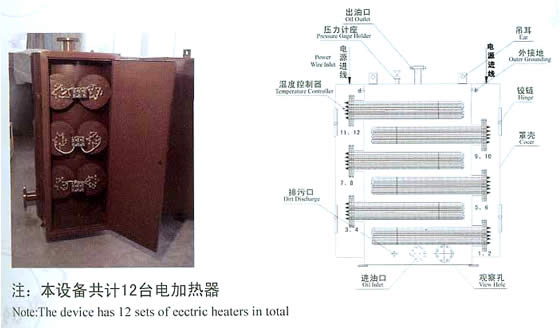• 防诈骗中心
• 客服中心 |
• 网站导航 |
• 设为主页 |
• 加入收藏
• 您当前位置： 首页> 产品库>江苏省>镇江市
相关分类：
• 湖南
• 长沙市
• 常德市
• 郴州市
• 衡阳市
• 怀化市
• 娄底市
• 邵阳市
• 湘潭市
• 湘西土家族苗族自治州
• 益阳市
• 永州市
• 岳阳市
• 张家界市
• 株洲市
• 山西
• 长治市
• 大同市
• 晋城市
• 晋中市
• 临汾市
• 吕梁市
• 朔州市
• 太原市
• 忻州市
• 阳泉市
• 运城市
• 安徽
• 安庆市
• 蚌埠市
• 亳州市
• 巢湖市
• 池州市
• 滁州市
• 阜阳市
• 合肥市
• 淮北市
• 淮南市
• 黄山市
• 六安市
• 马鞍山市
• 宿州市
• 铜陵市
• 芜湖市
• 宣城市
• 广西
• 百色市
• 北海市
• 崇左市
• 防城港市
• 贵港市
• 桂林市
• 河池市
• 贺州市
• 来宾市
• 柳州市
• 南宁市
• 钦州市
• 梧州市
• 玉林市
• 河南
• 安阳市
• 鹤壁市
• 焦作市
• 开封市
• 洛阳市
• 漯河市
• 南阳市
• 平顶山市
• 濮阳市
• 三门峡市
• 商丘市
• 新乡市
• 信阳市
• 许昌市
• 郑州市
• 周口市
• 驻马店市
• 吉林
• 白城市
• 白山市
• 长春市
• 吉林市
• 辽源市
• 四平市
• 松原市
• 通化市
• 延边朝鲜族自治州
• 广东
• 潮州市
• 东莞市
• 佛山市
• 广州市
• 河源市
• 惠州市
• 江门市
• 揭阳市
• 茂名市
• 梅州市
• 清远市
• 汕头市
• 汕尾市
• 韶关市
• 深圳市
• 阳江市
• 云浮市
• 湛江市
• 肇庆市
• 中山市
• 珠海市
• 辽宁
• 鞍山市
• 本溪市
• 朝阳市
• 大连市
• 丹东市
• 抚顺市
• 阜新市
• 葫芦岛市
• 锦州市
• 辽阳市
• 盘锦市
• 沈阳市
• 铁岭市
• 营口市
• 湖北
• 鄂州市
• 恩施土家族苗族自治州
• 黄冈市
• 黄石市
• 荆门市
• 荆州市
• 直辖行政单位
• 十堰市
• 随州市
• 武汉市
• 咸宁市
• 襄阳市
• 孝感市
• 宜昌市
• 江西
• 抚州市
• 赣州市
• 吉安市
• 景德镇市
• 九江市
• 南昌市
• 萍乡市
• 上饶市
• 新余市
• 宜春市
• 鹰潭市
• 浙江
• 杭州市
• 湖州市
• 嘉兴市
• 金华市
• 丽水市
• 宁波市
• 衢州市
• 绍兴市
• 台州市
• 温州市
• 舟山市
• 青海
• 果洛藏族自治州
• 海北藏族自治州
• 海东地区
• 海南藏族自治州
• 海西蒙古族藏族自治州
• 黄南藏族自治州
• 西宁市
• 玉树藏族自治州
• 甘肃
• 白银市
• 定西市
• 甘南藏族自治州
• 嘉峪关市
• 金昌市
• 酒泉市
• 兰州市
• 临夏回族自治州
• 陇南市
• 平凉市
• 庆阳市
• 天水市
• 武威市
• 张掖市
• 贵州
• 安顺市
• 毕节市
• 贵阳市
• 六盘水市
• 黔东南苗族侗族自治州
• 黔南布依族苗族自治州
• 黔西南布依族苗族自治州
• 铜仁地区
• 遵义市
• 陕西
• 安康市
• 宝鸡市
• 汉中市
• 商洛市
• 铜川市
• 渭南市
• 西安市
• 咸阳市
• 延安市
• 榆林市
• 西藏
• 阿里地区
• 昌都地区
• 拉萨市
• 林芝地区
• 那曲地区
• 日喀则地区
• 山南地区
• 宁夏
• 固原市
• 石嘴山市
• 吴忠市
• 银川市
• 中卫市
• 福建
• 福州市
• 龙岩市
• 南平市
• 宁德市
• 莆田市
• 泉州市
• 三明市
• 厦门市
• 漳州市
• 内蒙古
• 阿拉善盟
• 巴彦淖尔市
• 包头市
• 赤峰市
• 鄂尔多斯市
• 呼和浩特市
• 呼伦贝尔市
• 通辽市
• 乌海市
• 乌兰察布市
• 锡林郭勒盟
• 兴安盟
• 云南
• 保山市
• 楚雄彝族自治州
• 大理白族自治州
• 德宏傣族景颇族自治州
• 迪庆藏族自治州
• 红河哈尼族彝族自治州
• 昆明市
• 丽江市
• 临沧市
• 怒江傈僳族自治州
• 曲靖市
• 思茅市
• 文山壮族苗族自治州
• 西双版纳傣族自治州
• 玉溪市
• 昭通市
• 新疆
• 阿克苏地区
• 阿勒泰地区
• 巴音郭楞蒙古自治州
• 博尔塔拉蒙古自治州
• 昌吉回族自治州
• 哈密地区
• 和田地区
• 喀什地区
• 克拉玛依市
• 克孜勒苏柯尔克孜自治州
• 直辖行政单位
• 塔城地区
• 吐鲁番地区
• 乌鲁木齐市
• 伊犁哈萨克自治州
• 黑龙江
• 大庆市
• 大兴安岭地区
• 哈尔滨市
• 鹤岗市
• 黑河市
• 鸡西市
• 佳木斯市
• 牡丹江市
• 七台河市
• 齐齐哈尔市
• 双鸭山市
• 绥化市
• 伊春市
• 香港
• 香港
• 九龙
• 新界
• 澳门
• 澳门
• 其它地区
• 台湾
• 台中市
• 台南市
• 高雄市
• 台北市
• 基隆市
• 嘉义市
•3VBS210堆高车电池_睿博电源性价比高的堆高车蓄电池_你的理想选择

品牌:睿博牌,百思特,

出厂地:江苏省  镇江市

报价：面议

江苏睿博电源有限公司

黄金会员：主营：叉车电池,搬运车电池,堆高车电池,旅游观光车电池,免维护电池

•报价：面议

江苏普菲克电气科技有限公司

黄金会员：主营：动态电压恢复装置（PDVR）,静止型无功发生装置,无功补偿,电力电子式线路快速切...

•耐蚀合金|镇江供应质量硬的_耐蚀合金

品牌:天华特种钢,,

出厂地:江苏省  镇江市

报价：面议

镇江天华不锈钢制品有限公司

黄金会员：主营：马氏体时效钢,软磁不锈钢,18Ni300,4105IL,13-8MoPH

•电加热器代理商-江苏导热油电加热器供应

品牌:春鹏,,

出厂地:江苏省  镇江市

报价：面议

镇江市春鹏电器有限公司

黄金会员：主营：电加热器,控温柜,开关柜,桥架,母线

•灯具散热器批发-镇江质量良好的节能led散热器哪里买

品牌:奥星,,

出厂地:江苏省  镇江市

报价：面议

镇江奥星电子有限公司

黄金会员：主营：电子散热器,插片散热器,型材散热器,铝型材散热器,机箱散热器

•报价：面议

镇江市天祥精密机电有限公司

黄金会员：主营：高频焊机,熔炼炉,熔铜炉,中频熔炼炉,高频感应熔炼炉

•临沂储罐式电加热器 买储罐式电加热器就选裕太防爆

品牌:裕太,,

出厂地:江苏省  镇江市

报价：面议

镇江市裕太防爆电加热器有限公司

黄金会员：主营：电加热器,防爆电加热器,船用防爆电加热器,氢气电加热器,导热油炉电加热器

•密炼机配件供应商-大量供应品质可靠的密炼机配件

品牌:瑞阳,,

出厂地:江苏省  镇江市

报价：面议

丹阳市瑞阳橡塑机械有限公司

黄金会员：主营：开炼机,硫化机,密炼机,切胶机,挤出

•报价：面议

句容市茅山镇凌家园苗业种植基地

黄金会员：主营：榉树,朴树,乌桕,水杉,池杉

•推荐四氟管-知名厂家为您推荐高品质聚乙烯四氟管

品牌:威宇,,

出厂地:江苏省  镇江市

报价：面议

扬中市威宇氟塑制品有限公司

黄金会员：主营：聚四氟乙烯（F4）板,模压板,聚四氟乙烯制品,聚全氟乙丙烯,氟橡胶“O”形圈

• 没有找到合适的镇江市供应商？您可以发布采购信息

没有找到满足要求的镇江市供应商？您可以搜索 批发 公司

### 最新入驻厂家

相关产品:
3VBS210堆高车电池 动态电压恢复装置(PDVR)供货厂家 耐蚀合金 电加热器代理商 灯具散热器批发 口碑好的中频感应加热伟德betvictor99 临沂储罐式电加热器 密炼机配件供应商 落羽杉种植基地 推荐四氟管